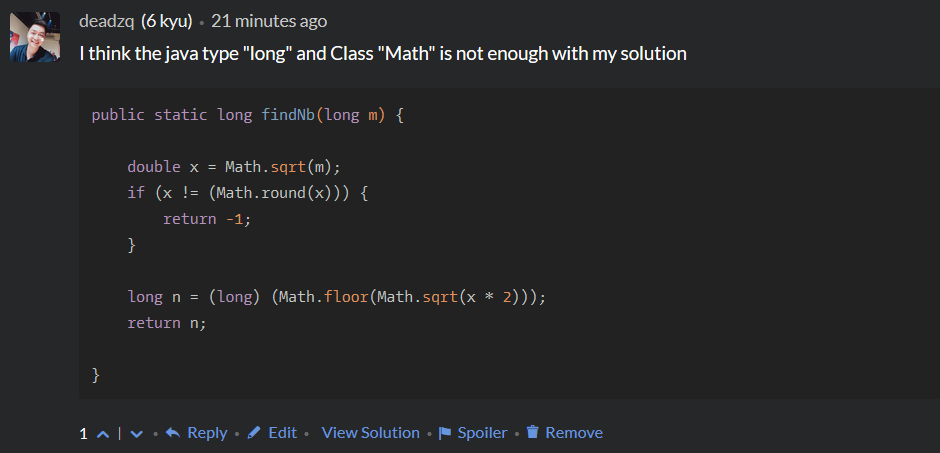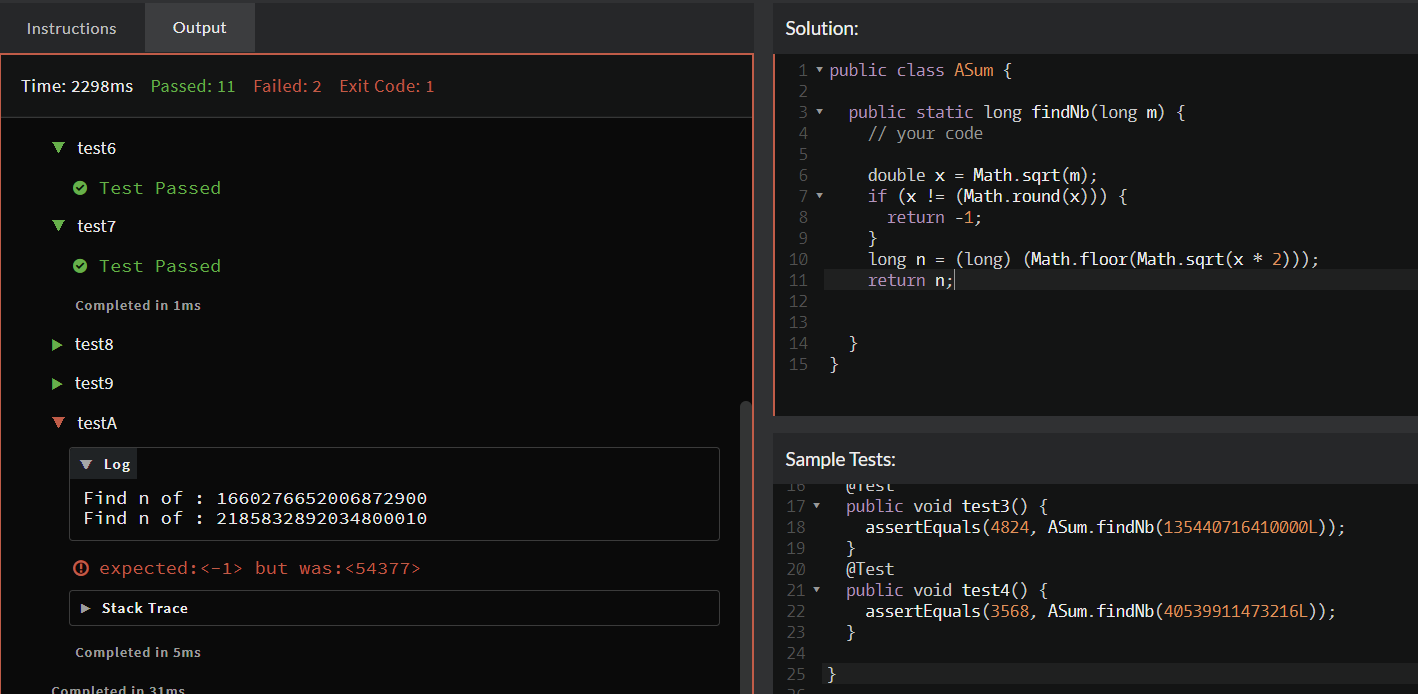#0
0
01. 云栖社区>
2. 博客>
3. 正文

## 同一个问题有多个解

ykmimi.com 2018-08-26 14:16:00 浏览767

https://www.codewars.com/kata/5592e3bd57b64d00f3000047

```public static long findNb0(long m) {
long n = 0;// 求得的n
long sum = 0;// 累加值与m进行判定
long s = 0;
boolean rightN = false;
while (s < m) {
sum += (s * s * s);

if (sum == m) {
rightN = true;
n = s;
break;
}
if (sum > m) {
break;
}

s++;

}
if (rightN) {
return n;

} else {
return -1;
}
}```

```    public static long findNb2(long m) {
long mm = 0, n = 0;
while (mm < m)
mm += ++n * n * n;
return mm == m ? n : -1;
}```

```public static long findNb(long m) {

double x = Math.sqrt(m); << 问题出在这里,应该为有小数的结果会可能返回正整数
if (x != (Math.round(x))) {
return -1;
}

long n = (long) (Math.floor(Math.sqrt(x * 2)));
return n;

}```

`long m = 1667089848622288897L;`

```......
......
......
1666827415525457025
1666958628200550400
1667089848622288896
1667221076790977409
-1```

>> 也许是java中的Math类计算失误(对于过大数值)

>> 或者是计算机底层的相乘出错了?(相乘使用的其实是加法),

>> 也许是我写的解答就是不对的?(对于有整型平方根的整数,其是有1³+2³+3³+......+n³的和一样的整数的这件事情,我觉得应该没问题,毕竟测试通过率为98%(当然除了这个数值和其它更大数值))-------------------------------------------------------长长的分割线,记录下我对家的思念--------------------------------------------------------------ykmimi.com
+ 关注